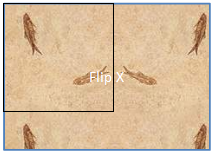# `<tile>` (Tile)

This element specifies that a BLIP should be tiled to fill the available space. This element defines a "tile" rectangle within the bounding box. The image is encompassed within the tile rectangle, and the tile rectangle is tiled across the bounding box to fill the entire area.

example:

The following is a fill with the flip attribute set to "x". The black interior rectangle indicates the tile rectangle. Notice that the adjacent rectangle to the right in the tile has been flipped along the x-axis.Parent Elements

`<blipFill>`5.8.2.2); `<blipFill>`5.1.10.14); `<blipFill>`5.6.2.2); `<blipFill>`5.2.2.1); `<blipFill>`4.4.1.4)

Attributes

Description

`<algn>` (Alignment)

Specifies where to align the first tile with respect to the shape. Alignment happens after the scaling, but before the additional offset.

The possible values for this attribute are defined by the ST_RectAlignment simple type (§5.1.12.53).

`<flip>` (Tile Flipping)

Specifies the direction(s) in which to flip the source image while tiling. Images may be flipped horizontally, vertically, or in both directions to fill the entire region.

The possible values for this attribute are defined by the ST_TileFlipMode simple type (§5.1.12.86).

`<sx>` (Horizontal Ratio)

Specifies the amount to horizontally scale the srcRect.

The possible values for this attribute are defined by the ST_Percentage simple type (§5.1.12.41).

`<sy>` (Vertical Ratio)

Specifies the amount to vertically scale the srcRect.

The possible values for this attribute are defined by the ST_Percentage simple type (§5.1.12.41).

`<tx>` (Horizontal Offset)

Specifies additional horizontal offset after alignment.

The possible values for this attribute are defined by the ST_Coordinate simple type (§5.1.12.16).

`<ty>` (Vertical Offset)

Specifies additional vertical offset after alignment.

The possible values for this attribute are defined by the ST_Coordinate simple type (§5.1.12.16).

The following XML Schema fragment defines the contents of this element:

``````<complexType name="CT_TileInfoProperties">
<attribute name="tx" type="ST_Coordinate" use="optional"/>
<attribute name="ty" type="ST_Coordinate" use="optional"/>
<attribute name="sx" type="ST_Percentage" use="optional"/>
<attribute name="sy" type="ST_Percentage" use="optional"/>
<attribute name="flip" type="ST_TileFlipMode" use="optional"/>
<attribute name="algn" type="ST_RectAlignment" use="optional"/>
</complexType>``````